Science, Maths & Technology

### Become an OU studentUsing a scientific calculator

Start this free course now. Just create an account and sign in. Enrol and complete the course for a free statement of participation or digital badge if available.

# 8 Finding angles from trigonometric ratios

Inverse trigonometric values can be found using the second functions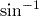,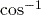and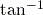of the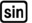,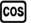and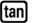keys. These functions are used in a similar manner to,and.

## Activity 17 Finding angles from trigonometric ratios

Calculate the value of each of the following expressions using your calculator, where possible, giving your answers correct to 1 decimal place.

1.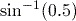2.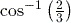3.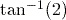4.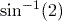1.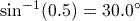.
If you obtained the answer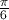or 0.5236 (to 4 decimal places), then your calculator is set to work in radians. Make sure that you are working in degrees.
2.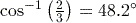(to 1 decimal place).
3.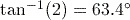(to 1 decimal place).
4. The calculationis not possible, and your calculator will give a ‘Math Error’. There is no angle whose sine is 2, because the hypotenuse is always the largest side of a right-angled triangle, and hence the maximum value the sine of an angle can be is 1.